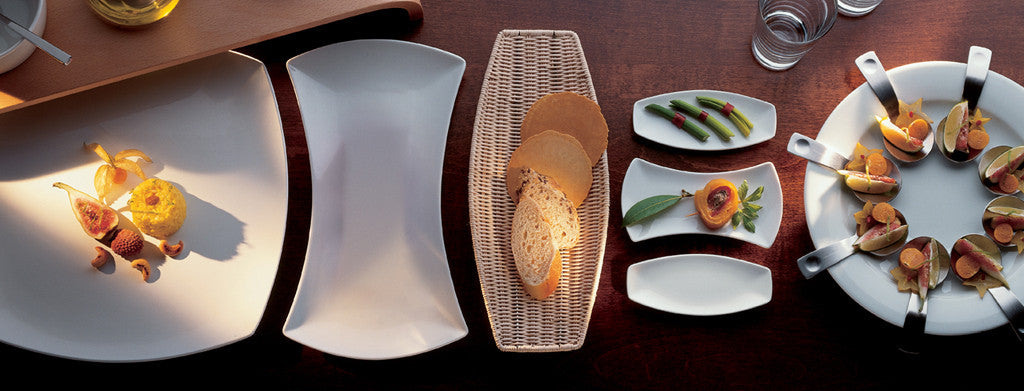Shopping Cart

### Refine

View all

#### Price# Crockery£17.40 each (ex VAT)£22.09 each (ex VAT)£10.79 each (ex VAT)£8.88 each (ex VAT)£34.94 each (ex VAT)£22.09 each (ex VAT)£18.12 each (ex VAT)£8.53 each (ex VAT)£7.66 each (ex VAT)£16.87 each (ex VAT)£9.46 each (ex VAT)£12.26 each (ex VAT)£9.46 each (ex VAT)£19.55 each (ex VAT)£20.52 each (ex VAT)£13.79 each (ex VAT)£8.40 each (ex VAT)£22.83 each (ex VAT)£19.55 each (ex VAT)£9.82 each (ex VAT)£19.55 each (ex VAT)£16.92 each (ex VAT)£13.08 each (ex VAT)£9.26 each (ex VAT)£6.96 each (ex VAT)£12.26 each (ex VAT)£34.94 each (ex VAT)£34.94 each (ex VAT)£25.81 each (ex VAT)£21.03 each (ex VAT)£10.67 each (ex VAT)£8.14 each (ex VAT)£25.81 each (ex VAT)£21.03 each (ex VAT)£14.90 each (ex VAT)£12.73 each (ex VAT)£7.66 each (ex VAT)£24.00 each (ex VAT)£17.40 each (ex VAT)£14.17 each (ex VAT)£13.08 each (ex VAT)£6.96 each (ex VAT)